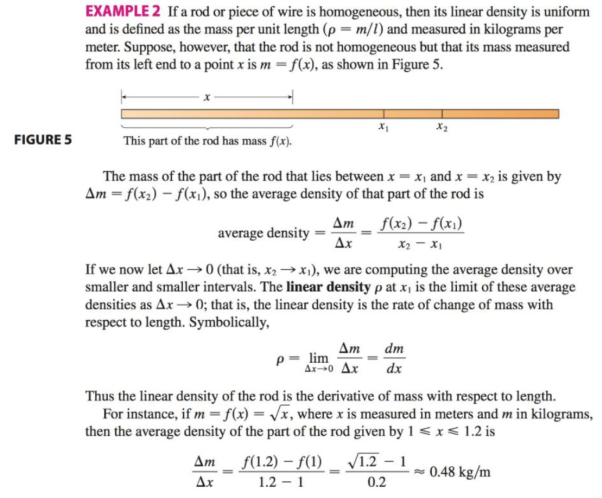Chapter 3.7, Problem 17E### Single Variable Calculus: Early Tr...

8th Edition
James Stewart
ISBN: 9781305270343

#### Solutions

Chapter
Section### Single Variable Calculus: Early Tr...

8th Edition
James Stewart
ISBN: 9781305270343
Textbook Problem

# The mass of the part of a metal rod that lies between its left end and a point x meters to the right is 3x2 kg. Find the linear density {see Example 2) when x is (a) 1m, (b) 2m, and (c) 3m. Where is the density the highest? The lowest?EXAMPLE 2(a)

To determine

To find: The linear density at x=1m.

Explanation

Given:

The mass of the part of the metal rod which lies between its left end and a point x m away from the right end is 3x2kg.

Calculation:

The mass of the metal rod is, m(x)=3x2

(b)

To determine

To find: The linear density at x=2m.

(c)

To determine

To find: The linear density at x=3m; and to tell the conclusion obtained from the parts (a), (b) and (c).

### Still sussing out bartleby?

Check out a sample textbook solution.

See a sample solution

#### The Solution to Your Study Problems

Bartleby provides explanations to thousands of textbook problems written by our experts, many with advanced degrees!

Get Started

#### Expand each expression in Exercises 122. (2x3)2

Finite Mathematics and Applied Calculus (MindTap Course List)

#### Evaluate Evaluate the expression. 10. 5|104|

Precalculus: Mathematics for Calculus (Standalone Book)

#### f(x)1x(x+2)(x3)

Applied Calculus for the Managerial, Life, and Social Sciences: A Brief Approach

#### In Problems , find and.

Mathematical Applications for the Management, Life, and Social Sciences

#### sec1(23)= a) 3 b) 3 c) 6 d) 6

Study Guide for Stewart's Single Variable Calculus: Early Transcendentals, 8th